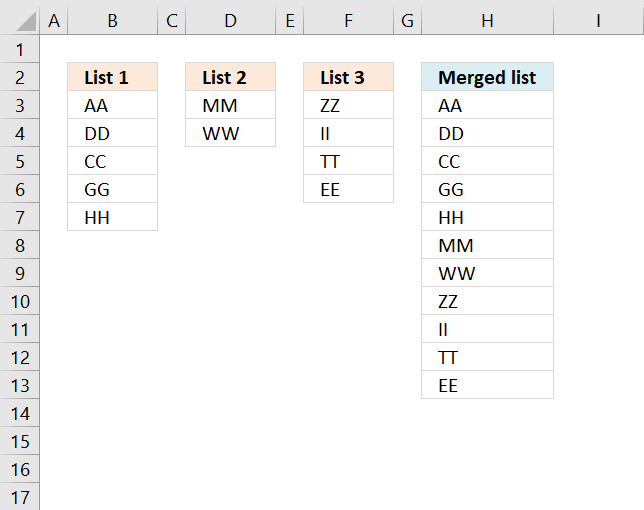Author: Oscar Cronquist Article last updated on November 20, 2018The above image demonstrates a formula that adds values in three different columns into one column.

Formula in H2:

=IFERROR(INDEX(\$B\$3:\$B\$7, ROWS(H2:\$H\$2)), IFERROR(INDEX(\$D\$3:\$D\$4, ROWS(H2:\$H\$2)-ROWS(\$B\$3:\$B\$7)), IFERROR(INDEX(\$F\$3:\$F\$6, ROWS(H2:\$H\$2)-ROWS(\$B\$3:\$B\$7)-ROWS(\$D\$3:\$D\$4)), "")))

Copy cell H2 and paste to cells below.

### Explaining formula in cell H2

The IFERROR function moves the calculation to the next part (formula2) when the first part (formula1) begins to return errors. That is also true for the second part (formula2), when errors occur the calculation continues with the third part (formula3)

IFERROR(IFERROR(formula1, formula2), formula3)

Formula1 extracts values from List1. Formula2 extracts values from List2. Formula3 extracts values from List3.

#### Step 1 - Count cells vertically

The ROWS function counts rows in a cell reference. H2:\$H\$2 is special, it expands as the formula is copied to cells below.

ROWS(H2:\$H\$2)

returns 1.

#### Step 2 - Return value

The INDEX function returns values from a cell range based on a row number and column number.

INDEX(\$B\$3:\$B\$7, ROWS(H2:\$H\$2))

becomes

INDEX(\$B\$3:\$B\$7, 1)

becomes

INDEX({"AA";"DD";"CC";"GG";"HH"}, 1)

and returns "AA" in cell H3.

#### Step 3 - Loop

When the formula starts returning errors the second part of the formula begins.

INDEX(\$D\$3:\$D\$4, ROWS(H2:\$H\$2)-ROWS(\$B\$3:\$B\$7))

It also takes into account the number of values returned from the first cell range, for example in cell H8:

INDEX(\$D\$3:\$D\$4, ROWS(H7:\$H\$2)-ROWS(\$B\$3:\$B\$7))

becomes

INDEX(\$D\$3:\$D\$4, 6-ROWS(\$B\$3:\$B\$7))

becomes

INDEX(\$D\$3:\$D\$4, 6-5)

becomes

INDEX({"MM";"WW"}, 1)

and returns "MM" in cell H8.

### Excel 2003 and earlier versions:

=INDEX((List1, List2, List3), ROW(A1)*(ROW(A1)<=ROWS(List1))+(ROW(A1)-ROWS(List1))*(ROW(A1)>ROWS(List1))*(ROW(A1)<=(ROWS(List1)+ROWS(List2)))+(ROW(A1)-ROWS(List1)-ROWS(List2))*(ROW(A1)>ROWS(List1))*(ROW(A1)>(ROWS(List1)+ROWS(List2)))*(ROW(A1)<=(ROWS(List1)+ROWS(List2)+ROWS(List3))), , (ROW(A1)<=ROWS(List1))*1+(ROW(A1)>ROWS(List1))*(ROW(A1)<=(ROWS(List1)+ROWS(List2)))*2+(ROW(A1)>ROWS(List1))*(ROW(A1)>(ROWS(List1)+ROWS(List2)))*(ROW(A1)<=(ROWS(List1)+ROWS(List2)+ROWS(List3)))*3) + ENTER

Named ranges
List1 (A2:A6)
List2 (B2:B3)
List3 (C2:C5)